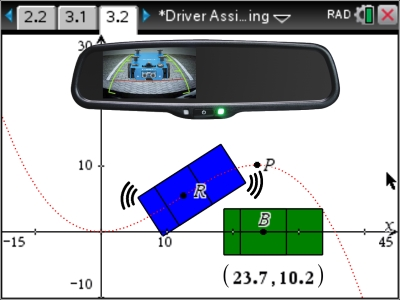•• ##### Device
•TI-Nspire™ CX CAS
•TI-Nspire™ CX

4.5

# QLD (Nspire): Self Parking Car

by Texas Instruments#### Objectives

• Transformations of a trigonometric function
• Transformations of a polynomial function
• Derivative of a polynomial
• Anti-derivative of a polynomial
• Simultaneous equations
• Distance between a point and function
• Define and build a hybrid (piecewise) function

#### Vocabulary

• Differentiable
• Continuous
• Point of inflexion
• Derivative
• Integral
• Hybrid or Piecewise
• Join smoothly = equal gradients